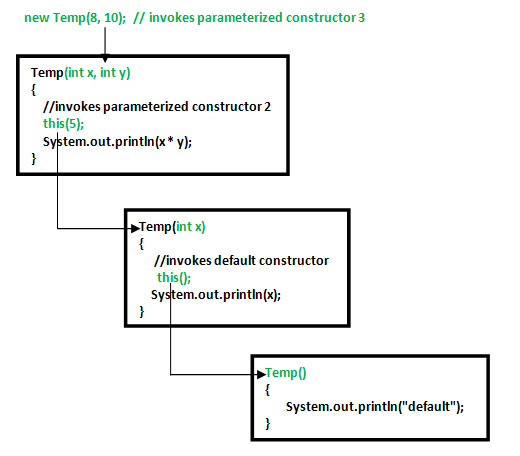# Constructor Chaining In Java with Examples

• Difficulty Level : Easy
• Last Updated : 16 Aug, 2021

Prerequisite – Constructors in Java
Constructor chaining is the process of calling one constructor from another constructor with respect to current object.
Constructor chaining can be done in two ways:

• Within same class: It can be done using this() keyword for constructors in same class
• From base class: by using super() keyword to call constructor from the base class.

Constructor chaining occurs through inheritance. A sub class constructor’s task is to call super class’s constructor first. This ensures that creation of sub class’s object starts with the initialization of the data members of the super class. There could be any numbers of classes in inheritance chain. Every constructor calls up the chain till class at the top is reached.
Why do we need constructor chaining ?
This process is used when we want to perform multiple tasks in a single constructor rather than creating a code for each task in a single constructor we create a separate constructor for each task and make their chain which makes the program more readable.

Constructor Chaining within same class using this() keyword :## Java

 `// Java program to illustrate Constructor Chaining` `// within same class Using this() keyword` `class` `Temp` `{` `    ``// default constructor 1` `    ``// default constructor will call another constructor` `    ``// using this keyword from same class` `    ``Temp()` `    ``{` `        ``// calls constructor 2` `        ``this``(``5``);` `        ``System.out.println(``"The Default constructor"``);` `    ``}`   `    ``// parameterized constructor 2` `    ``Temp(``int` `x)` `    ``{` `        ``// calls constructor 3` `        ``this``(``5``, ``15``);` `        ``System.out.println(x);` `    ``}`   `    ``// parameterized constructor 3` `    ``Temp(``int` `x, ``int` `y)` `    ``{` `        ``System.out.println(x * y);` `    ``}`   `    ``public` `static` `void` `main(String args[])` `    ``{` `        ``// invokes default constructor first` `        ``new` `Temp();` `    ``}` `}`

Output:

```75
5
The Default constructor```

Rules of constructor chaining :

1. The this() expression should always be the first line of the constructor.
2. There should be at-least be one constructor without the this() keyword (constructor 3 in above example).
3. Constructor chaining can be achieved in any order.

What happens if we change the order of constructors?
Nothing, Constructor chaining can be achieved in any order

## Java

 `// Java program to illustrate Constructor Chaining` `// within same class Using this() keyword` `// and changing order of constructors` `class` `Temp` `{` `    ``// default constructor 1` `    ``Temp()` `    ``{` `        ``System.out.println(``"default"``);` `    ``}`   `    ``// parameterized constructor 2` `    ``Temp(``int` `x)` `    ``{` `        ``// invokes default constructor` `        ``this``();` `        ``System.out.println(x);` `    ``}`   `    ``// parameterized constructor 3` `    ``Temp(``int` `x, ``int` `y)` `    ``{` `        ``// invokes parameterized constructor 2` `        ``this``(``5``);` `        ``System.out.println(x * y);` `    ``}`   `    ``public` `static` `void` `main(String args[])` `    ``{` `        ``// invokes parameterized constructor 3` `        ``new` `Temp(``8``, ``10``);` `    ``}` `}`

Output:

```default
5
80```

NOTE: In example 1, default constructor is invoked at the end, but in example 2 default constructor is invoked at first. Hence, order in constructor chaining is not important.

Constructor Chaining to other class using super() keyword :

## Java

 `// Java program to illustrate Constructor Chaining to` `// other class using super() keyword` `class` `Base` `{` `    ``String name;`   `    ``// constructor 1` `    ``Base()` `    ``{` `        ``this``(``""``);` `        ``System.out.println(``"No-argument constructor of"` `+ ` `                                           ``" base class"``);` `    ``}`   `    ``// constructor 2` `    ``Base(String name)` `    ``{` `        ``this``.name = name;` `        ``System.out.println(``"Calling parameterized constructor"` `                                              ``+ ``" of base"``);` `    ``}` `}`   `class` `Derived ``extends` `Base` `{` `    ``// constructor 3` `    ``Derived()` `    ``{` `        ``System.out.println(``"No-argument constructor "` `+ ` `                           ``"of derived"``);` `    ``}`   `    ``// parameterized constructor 4` `    ``Derived(String name)` `    ``{` `        ``// invokes base class constructor 2` `        ``super``(name);` `        ``System.out.println(``"Calling parameterized "` `+ ` `                           ``"constructor of derived"``);` `    ``}`   `    ``public` `static` `void` `main(String args[])` `    ``{` `        ``// calls parameterized constructor 4` `        ``Derived obj = ``new` `Derived(``"test"``);`   `        ``// Calls No-argument constructor` `        ``// Derived obj = new Derived();` `    ``}` `}`

Output:

```Calling parameterized constructor of base
Calling parameterized constructor of derived```

Note : Similar to constructor chaining in same class, super() should be the first line of the constructor as super class’s constructor are invoked before the sub class’s constructor.
Alternative method : using Init block
When we want certain common resources to be executed with every constructor we can put the code in the init block. Init block is always executed before any constructor, whenever a constructor is used for creating a new object.
Example 1:

## Java

 `class` `Temp` `{` `    ``// block to be executed before any constructor.` `    ``{` `        ``System.out.println(``"init block"``);` `    ``}`   `    ``// no-arg constructor` `    ``Temp()` `    ``{` `        ``System.out.println(``"default"``);` `    ``}`   `    ``// constructor with one argument.` `    ``Temp(``int` `x)` `    ``{` `        ``System.out.println(x);` `    ``}`   `    ``public` `static` `void` `main(String[] args)` `    ``{` `        ``// Object creation by calling no-argument ` `        ``// constructor.` `        ``new` `Temp();`   `        ``// Object creation by calling parameterized ` `        ``// constructor with one parameter.` `        ``new` `Temp(``10``);` `    ``}` `}`

Output:

```init block
default
init block
10```

NOTE: If there are more than one blocks, they are executed in the order in which they are defined within the same class. See the ex.
Example :

## Java

 `class` `Temp` `{` `    ``// block to be executed first` `    ``{` `        ``System.out.println(``"init"``);` `    ``}` `    ``Temp()` `    ``{` `        ``System.out.println(``"default"``);` `    ``}` `    ``Temp(``int` `x)` `    ``{` `        ``System.out.println(x);` `    ``}`   `    ``// block to be executed after the first block` `    ``// which has been defined above.` `    ``{` `        ``System.out.println(``"second"``);` `    ``}` `    ``public` `static` `void` `main(String args[])` `    ``{` `        ``new` `Temp();` `        ``new` `Temp(``10``);` `    ``}` `}`

Output :

```init
second
default
init
second
10```

This article is contributed by Apoorva singh. If you like GeeksforGeeks and would like to contribute, you can also write an article using write.geeksforgeeks.org or mail your article to review-team@geeksforgeeks.org. See your article appearing on the GeeksforGeeks main page and help other Geeks.